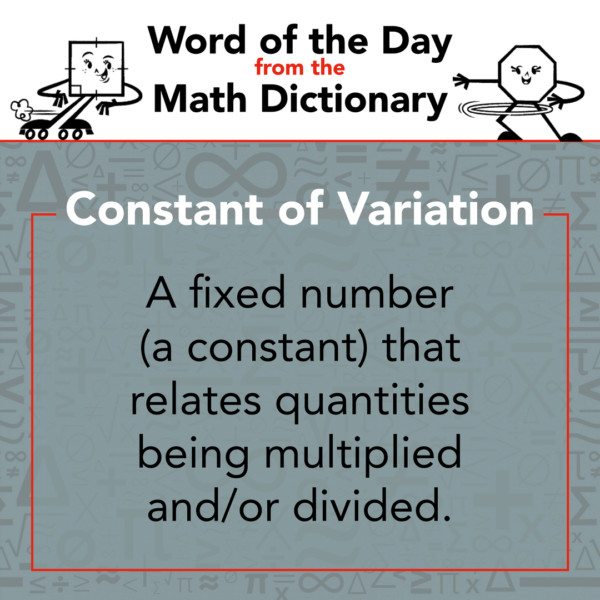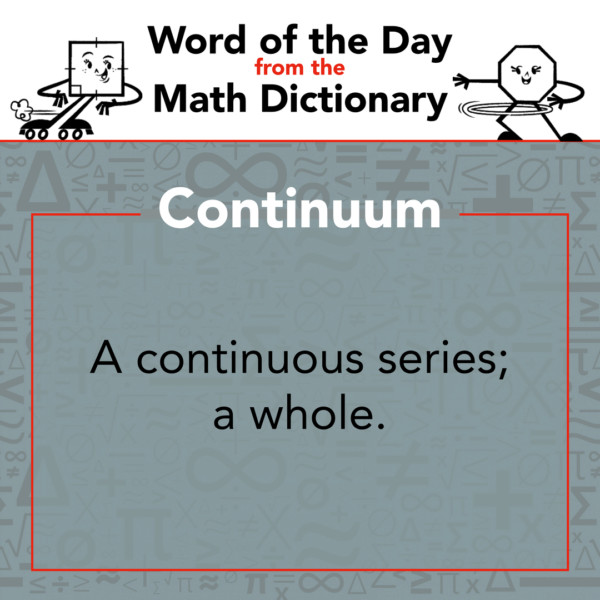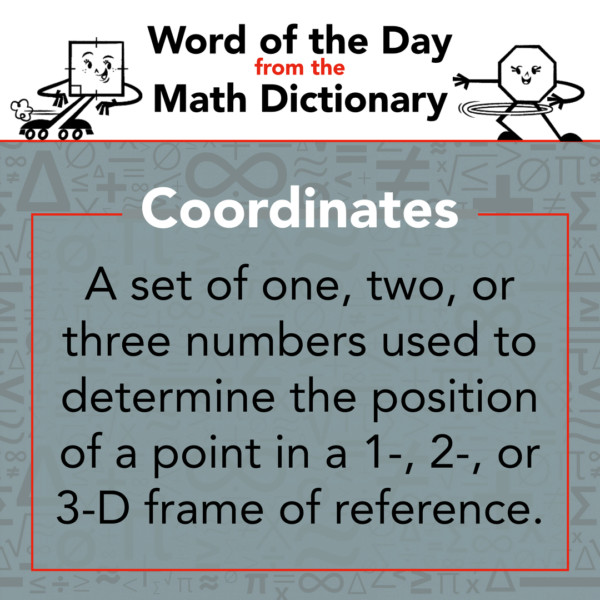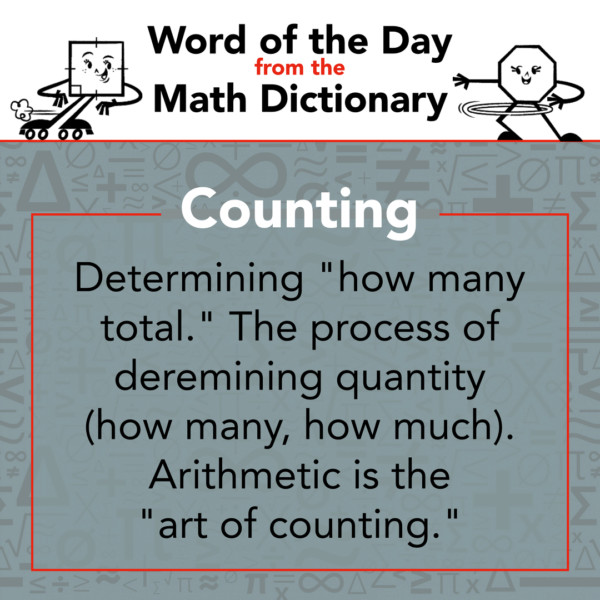877-601-6284
Get Started by Finding a Local Center

# Math Word of the Day - Week of November 22

Nov 22, 2020 | Location Frisco East

Math Word of the Day comes from the Math Dictionary written by Mathnasium.

### Constant of Variation

Sunday, November 22

A fixed number (a constant) that relates quantities being multiplied and/or divided.

Examples:

• In xy = 12, 12 is the constant of variation
• In x/y = 6 and x = 6y, 6 is the constant of variation
• In z/xy = 3 and z = 3xy, 3 is the constant of variation### Continuum

Monday, November 23

A continuous series; a whole.### Coordinates

Tuesday, November 24

A set of one, two, or three numbers used to determine the position of a point in a 1-, 2-, or 3-D frame of reference.### Coefficient of Correlation

Wednesday, November 25

A statistical measure that relates how well a set of data points can be modeled by a trend line.### Cosine

Thursday, November 26

The trigonometric function that is defined as the ratio of the leg adjacent to an angle to the hypotenuse of a right angle.### Counter Example

Friday, November 27

An example of a conditional statement in which the hypothesis is true and the conclusion is false.### Counting

Saturday, November 28

Determining "how many total." The process of determining quantity (how many, how much). Arithmetic is the "art of counting."##### Locations near
ME
Capitol Hill DC 28.48 mi
621 Pennsylvania Ave SE
1st-floor unit
Washington, DC 20003